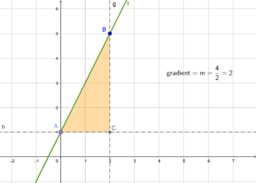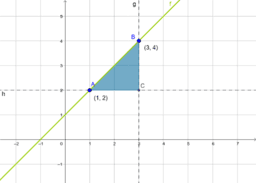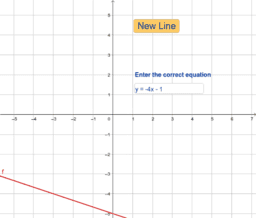# Linear functions

Introduction to linear functions, equations, slope (gradient) and y-intercept. In mathematics, a function assigns exactly one dependent variable (often y) to an independent variable (often x). A function might be given by an expression, e.g., y = 2x + 1, that states the rule in which each x-value determines its corresponding y-value
•### Slope

•### Gradient - 2 given points

•### Test yourself

•